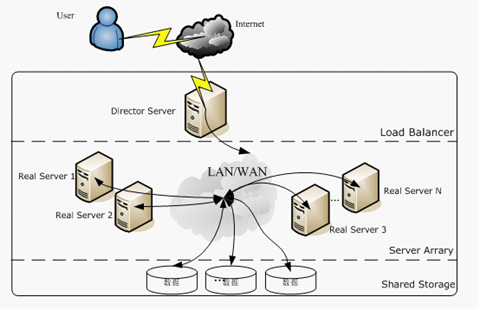# 机器学习算法之CatBoost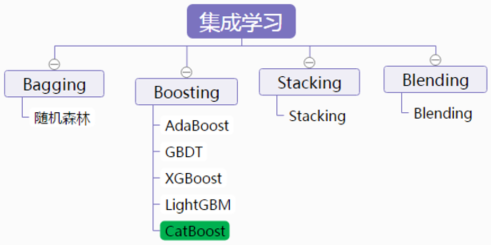## CatBoost简介

CatBoost这个名字来自两个词“Category”和“Boosting”。如前所述，该库可以很好地处理各种类别型数据，是一种能够很好地处理类别型特征的梯度提升算法库。

CatBoost的优点

• 性能卓越：在性能方面可以匹敌任何先进的机器学习算法
• 鲁棒性/强健性：它减少了对很多超参数调优的需求，并降低了过度拟合的机会，这也使得模型变得更加具有通用性
• 易于使用：提供与scikit集成的Python接口，以及R和命令行界面
• 实用：可以处理类别型、数值型特征
• 可扩展：支持自定义损失函数

CatBoost的开发人员将其性能与标准ML数据集的竞争对手进行了比较：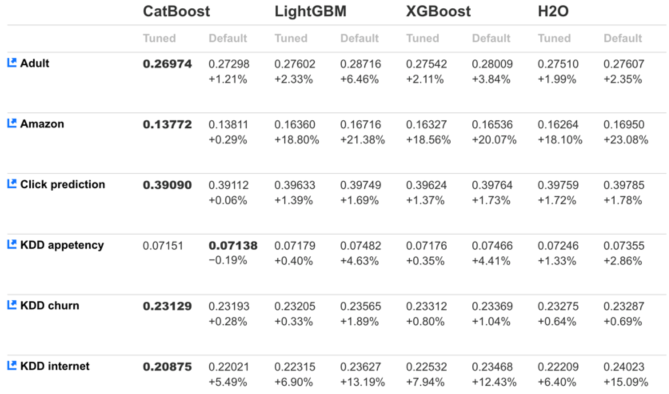## CatBoost技术介绍

### 类别型特征

CatBoost 算法的设计初衷是为了更好的处理 GBDT 特征中的 categorical features 。在处理 GBDT 特征中的 categorical features 的时候，最简单的方法是用 categorical feature 对应的标签的平均值来替换。在决策树中，标签平均值将作为节点分裂的标准。这种方法被称为 Greedy Target-based Statistics , 简称 Greedy TBS，用公式来表达就是：

$$\hat{x}_k^i=\frac{\sum_{j=1}^{n}[x_{j,k}=x_{i,k}]\cdot Y_i}{\sum_{j=1}^{n}[x_{j,k}=x_{i,k}]}$$

$$\hat{x}_k^i=\frac{\sum_{j=1}^{p-1}[x_{\sigma _j,k}=x_{\sigma _p,k}]Y_{\sigma _j}+a\cdot P}{\sum_{j=1}^{p-1}[x_{\sigma _j,k}=x_{\sigma _p,k}]+a}$$

CatBoost算法为了在每个步骤中拟合最优的先验条件，我们考虑多个先验条件，为每个先验条件构造一个特征，这在质量上比提到的标准技术更有效。

### 克服梯度偏差

CatBoost，和所有标准梯度提升算法一样，都是通过构建新树来拟合当前模型的梯度。然而，所有经典的提升算法都存在由有偏的点态梯度估计引起的过拟合问题。在每个步骤中使用的梯度都使用当前模型中的相同的数据点来估计，这导致估计梯度在特征空间的任何域中的分布与该域中梯度的真实分布相比发生了偏移，从而导致过拟合。

### 快速评分

CatBoost使用oblivious树作为基本预测器。在这类树中，相同的分割准则在树的整个级别上使用。这种树是平衡的，不太容易过拟合。梯度提升oblivious树被成功地用于各种学习任务。在oblivious树中，每个叶子节点的索引可以被编码为长度等于树深度的二进制向量。这在CatBoost模型评估器中得到了广泛的应用：我们首先将所有浮点特征、统计信息和独热编码特征进行二值化，然后使用二进制特征来计算模型预测值。

$$\sum_{i=0}^{d-1}2^{i}\cdot B(x,f(t,j))$$

### 基于GPU实现快速学习

• 存储二进制特征用1位，每个整数包括32个特征
• 存储不超过15个值的特征用4位，每个整数包括8个特征
• 存储其他特征用8位（不同值的个数最大是255），每个整数包括4个特征

CatBoost实现了多种处理类别型特征的方法。对于独热编码特征，我们不需要任何特殊处理，基于直方图的分割搜索方法可以很容易地处理这种情况。在数据预处理阶段，就可以对单个类别型特征进行统计计算。CatBoost还对特征组合使用统计信息。处理它们是算法中速度最慢、消耗内存最多的部分。

CatBoost使用完美哈希来存储类别特征的值，以减少内存使用。由于GPU内存的限制，我们在CPU RAM中存储按位压缩的完美哈希，以及要求的数据流、重叠计算和内存等操作。动态地构造特征组合要求我们为这个新特征动态地构建（完美）哈希函数，并为哈希的每个唯一值计算关于某些排列的统计数据。CatBoost使用基数排序来构建完美的哈希，并通过哈希来分组观察。在每个组中，需要计算一些统计量的前缀和。该统计量的计算使用分段扫描GPU图元进行（CatBoost分段扫描实现通过算子变换完成，并且基于CUB中扫描图元的高效实现）。

CatBoost中的GPU实现可支持多个GPU。分布式树学习可以通过数据或特征进行并行化。CatBoost采用多个学习数据集排列的计算方案，在训练期间计算分类特征的统计数据。

## CatBoost的安装与使用

CatBoost的安装非常的简单，只需执行pip install catboost即可。

### CatBoost使用示例

import numpy as np
import catboost as cb

train_data = np.random.randint(0, 100, size=(100, 10))
train_label = np.random.randint(0, 2, size=(100))
test_data = np.random.randint(0,100, size=(50,10))

model = cb.CatBoostClassifier(iterations=2, depth=2, learning_rate=0.5, loss_function='Logloss',
logging_level='Verbose')
model.fit(train_data, train_label, cat_features=[0,2,5])
preds_class = model.predict(test_data)
preds_probs = model.predict_proba(test_data)
print('class = ',preds_class)
print('proba = ',preds_probs)


• Fit
• X: 输入数据数据类型可以是，list; pandas.DataFrame; pandas.Series
• y=None
• cat_features=None: 拿来做处理的类别特征
• sample_weight=None: 输入数据的样本权重
• logging_level=None: 控制是否输出日志信息，或者何种信息
• plot=False: 训练过程中，绘制，度量值，所用时间等
• eval_set=None: 验证集合，数据类型list(X, y)tuples
• baseline=None
• use_best_model=None
• verbose=None
• predict 返回验证样本所属类别，数据类型为array
• predict_proba 返回验证样本所属类别的概率，数据类型为array
• get_feature_importance
• eval_metrics
• save_model
• get_params
• score

• is_fitted_
• tree_count_
• feature_importances_
• random_seed_

### CatBoost参数详解

• loss_function 损失函数，支持的有RMSE, Logloss, MAE, CrossEntropy, Quantile, LogLinQuantile, Multiclass, MultiClassOneVsAll, MAPE, Poisson。默认RMSE。
• custom_metric 训练过程中输出的度量值。这些功能未经优化，仅出于信息目的显示。默认None。
• eval_metric 用于过拟合检验（设置True）和最佳模型选择（设置True）的loss function，用于优化。
• iterations 最大树数。默认1000。
• learning_rate 学习率。默认03。
• random_seed 训练时候的随机种子
• l2_leaf_reg L2正则参数。默认3
• bootstrap_type 定义权重计算逻辑，可选参数：Poisson (supported for GPU only)/Bayesian/Bernoulli/No，默认为Bayesian
• bagging_temperature 贝叶斯套袋控制强度，区间[0, 1]。默认1。
• subsample 设置样本率，当bootstrap_type为Poisson或Bernoulli时使用，默认66
• sampling_frequency 设置创建树时的采样频率，可选值PerTree/PerTreeLevel，默认为PerTreeLevel
• random_strength 分数标准差乘数。默认1。
• use_best_model 设置此参数时，需要提供测试数据，树的个数通过训练参数和优化loss function获得。默认False。
• best_model_min_trees 最佳模型应该具有的树的最小数目。
• depth 树深，最大16，建议在1到10之间。默认6。
• ignored_features 忽略数据集中的某些特征。默认None。
• one_hot_max_size 如果feature包含的不同值的数目超过了指定值，将feature转化为float。默认False
• has_time 在将categorical features转化为numerical features和选择树结构时，顺序选择输入数据。默认False（随机）
• rsm 随机子空间（Random subspace method）。默认1。
• nan_mode 处理输入数据中缺失值的方法，包括Forbidden(禁止存在缺失)，Min(用最小值补)，Max(用最大值补)。默认Min。
• fold_permutation_block_size 数据集中的对象在随机排列之前按块分组。此参数定义块的大小。值越小，训练越慢。较大的值可能导致质量下降。
• leaf_estimation_iterations 计算叶子值时梯度步数。
• leaf_estimation_backtracking 在梯度下降期间要使用的回溯类型。
• fold_len_multiplier folds长度系数。设置大于1的参数，在参数较小时获得最佳结果。默认2。
• approx_on_full_history 计算近似值，False：使用1／fold_len_multiplier计算；True：使用fold中前面所有行计算。默认False。
• class_weights 类别的权重。默认None。
• scale_pos_weight 二进制分类中class 1的权重。该值用作class 1中对象权重的乘数。
• boosting_type 增压方案
• allow_const_label 使用它为所有对象训练具有相同标签值的数据集的模型。默认为False

CatBoost默认参数：

• ‘iterations’: 1000,
• ‘learning_rate’:0.03,
• ‘l2_leaf_reg’:3,
• ‘bagging_temperature’:1,
• ‘subsample’:0.66,
• ‘random_strength’:1,
• ‘depth’:6,
• ‘rsm’:1,
• ‘one_hot_max_size’:2
• ‘fold_len_multiplier’:2,
• ‘border_count’:128,

CatBoost参数取值范围：

• ‘learning_rate’:Log-uniform distribution [e^{-7}, 1]
• ‘random_strength’:Discrete uniform distribution over a set {1, 20}
• ‘one_hot_max_size’:Discrete uniform distribution over a set {0, 25}
• ‘l2_leaf_reg’:Log-uniform distribution [1, 10]
• ‘bagging_temperature’:Uniform [0, 1]
• ‘gradient_iterations’:Discrete uniform distribution over a set {1, 10}‘

### CatBoost参数调优

from catboost import CatBoostRegressor
from sklearn.model_selection import GridSearchCV

#指定category类型的列，可以是索引，也可以是列名
cat_features = [0,1,2,3,4,5,6,7,8,9,10,11,12,13]

X = df_ios_droped.iloc[:,:-1]
y = df_ios_droped.iloc[:,-1]

cv_params = {'iterations': [500,600,700,800]}
other_params = {
'iterations': 1000,
'learning_rate':0.03,
'l2_leaf_reg':3,
'bagging_temperature':1,
'random_strength':1,
'depth':6,
'rsm':1,
'one_hot_max_size':2,
'fold_len_multiplier':2,
'border_count':128,
}
model_cb = CatBoostRegressor(**other_params)
optimized_cb = GridSearchCV(estimator=model_cb, param_grid=cv_params, scoring='r2', cv=5, verbose=1, n_jobs=2)
optimized_cb.fit(X,y,cat_features =category_features)
print('参数的最佳取值：{0}'.format(optimized_cb.best_params_))
print('最佳模型得分:{0}'.format(optimized_cb.best_score_))
print(optimized_cb.cv_results_['mean_test_score'])
print(optimized_cb.cv_results_['params'])

# params = {'depth':[3,4,5,7,8,9,10],
#           'iterations':[100,250,500,1000],
#           'learning_rate':[0.001,0.01,0.003,0.1,0.2,0.3],
#           'l2_leaf_reg':[1,3,5,10,100],
#           'border_count':[2,4,8,16,32,64,128,256]}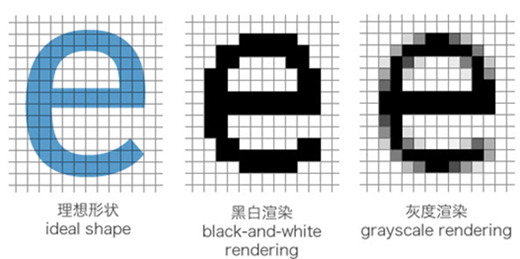##### 网站与APP开发中的字体设置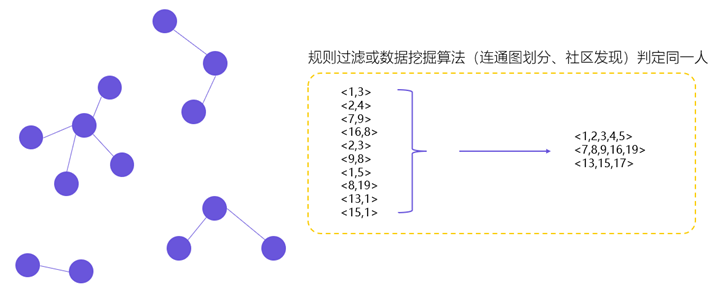##### 用户体系搭建之ID-Mapping